Next: Historical note Up: Lunar motion Previous: Precession of lunar perigee

# Regression of lunar ascending node

According to Equations (11.207) and (11.314), the mean ecliptic longitude of the lunar ascending node evolves in time as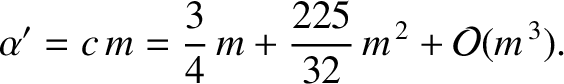(11.339)

where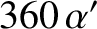(11.340)

Equation (11.339) implies that the ascending node regresses (i.e., its longitude decreases in time) at the mean rate of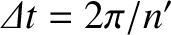degrees per year. As before, it is clear that this regression is entirely due to the perturbing influence of the Sun. Moreover, we find that the ascending node retreats by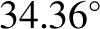degrees per year. Hence, we predict that the ascending node completes a full circuit about the Earth every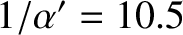years. In fact, the lunar ascending node completes a full circuit every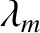years, so our prediction is fairly accurate.

Richard Fitzpatrick 2016-03-31